Notebook

# Alphalens Example Tear Sheet¶

Alphalens is designed to aid in the analysis of "alpha factors," data transformations that are used to predict future price movements of financial instruments. Alpha factors take the form of a single value for each asset on each day. The dimension of these values is not necessarily important. We evaluate an alpha factor by considering daily factor values relative to one another.

It is important to note the difference between an alpha factor and a trading algorithm. A trading algorithm uses an alpha factor, or combination of alpha factors to generate trades. Trading algorithms cover execution and risk constraints: the business of turning predictions into profits. Alpha factors, on the other hand, are focused soley on making predictions. This difference in scope lends itself to a difference in the methodologies used to evaluate alpha factors and trading algorithms. Alphalens does not contain analyses of things like transaction costs, capacity, or portfolio construction. Those interested in more implementation specific analyses are encouaged to check out pyfolio (https://github.com/quantopian/pyfolio), a library specifically geared towards the evaluation of trading algorithms.

In :
import numpy as np
import pandas as pd
from quantopian.research import run_pipeline
from quantopian.pipeline import Pipeline
from quantopian.pipeline.data.builtin import USEquityPricing
from quantopian.pipeline.factors import CustomFactor, Returns, AverageDollarVolume
from quantopian.pipeline.classifiers.morningstar import Sector

In :
universe_screen = AverageDollarVolume(window_length=20).top(500)

In :
pipe = Pipeline(
columns={
},
screen=universe_screen
)

In :
results = run_pipeline(pipe, '2015-06-30', '2016-06-30')
results = results.fillna(value=0.)

In :
momentum_factor = results["Momentum"]

Out:
2015-06-30 00:00:00+00:00  Equity(2 [AA])      -0.230704
Equity(24 [AAPL])    0.363660
Equity(62 [ABT])     0.224101
Equity(64 [ABX])    -0.402359
Name: Momentum, dtype: float64

The pricing data passed to alphalens should reflect the next available price after a factor value was observed at a given timestamp. The price must not be included in the calculation of the factor for that time. Always double check to ensure you are not introducing lookahead bias to your study.

In our example, before trading starts on 2014-12-2, we observe yesterday, 2014-12-1's factor value. The price we should pass to alphalens is the next available price after that factor observation: the open price on 2014-12-2.

In :
assets = results.index.levels.unique()
# We need to get a little more pricing data than the
# length of our factor so we can compare forward returns.
# We'll tack on another month in this example.
pricing = get_pricing(assets, start_date='2015-06-30', end_date='2016-07-31', fields='open_price')

In :
pricing.head()

Out:
Equity(2 [AA]) Equity(24 [AAPL]) Equity(62 [ABT]) Equity(64 [ABX]) Equity(67 [ADSK]) Equity(76 [TAP]) Equity(114 [ADBE]) Equity(122 [ADI]) Equity(128 [ADM]) Equity(154 [AEM]) ... Equity(49139 [FIT]) Equity(49141 [CPGX_WI]) Equity(49183 [WRK_WI]) Equity(49209 [BXLT]) Equity(49229 [KHC]) Equity(49242 [PYPL_V]) Equity(49506 [HPE_WI]) Equity(49515 [RACE]) Equity(49563 [SYF_WI]) Equity(49865 [HOT_WI])
2015-06-30 00:00:00+00:00 11.45 125.57 49.51 10.74 51.16 71.35 82.17 64.34 48.87 28.77 ... 35.05 28.67 55.380 NaN NaN NaN NaN NaN NaN NaN
2015-07-01 00:00:00+00:00 11.20 126.85 49.40 10.63 50.09 70.36 81.57 64.89 48.71 28.30 ... 39.29 29.40 54.847 NaN NaN NaN NaN NaN NaN NaN
2015-07-02 00:00:00+00:00 11.09 126.43 49.80 10.50 50.43 70.04 81.19 64.58 48.82 27.88 ... 41.82 NaN NaN 31.59 NaN NaN NaN NaN NaN NaN
2015-07-06 00:00:00+00:00 10.95 124.94 48.85 10.52 49.78 69.40 80.02 63.85 48.16 28.21 ... 41.15 29.56 57.565 30.80 71.00 NaN NaN NaN NaN NaN
2015-07-07 00:00:00+00:00 10.96 125.89 49.96 10.50 51.01 69.39 80.77 63.98 48.11 28.98 ... 42.36 30.85 57.710 31.70 73.99 37.73 NaN NaN NaN NaN

5 rows × 824 columns

Often, we'd want to know how our factor looks across various sectors. To generate sector level breakdowns, you'll need to pass alphalens a sector mapping for each traded name.

This mapping can come in the form of a MultiIndexed Series (with the same date/symbol index as your factor value) if you want to provide a sector mapping for each symbol on each day.

If you'd like to use constant sector mappings, you may pass symbol to sector mappings as a dict.

If your sector mappings come in the form of codes (as they do in this tutorial), you may also pass alphalens a dict of sector names to use in place of sector codes.

In :
MORNINGSTAR_SECTOR_CODES = {
-1: 'Misc',
101: 'Basic Materials',
102: 'Consumer Cyclical',
103: 'Financial Services',
104: 'Real Estate',
205: 'Consumer Defensive',
206: 'Healthcare',
207: 'Utilities',
308: 'Communication Services',
309: 'Energy',
310: 'Industrials',
311: 'Technology' ,
}

In :
sectors = results["Sector"]


# Importing Alphalens¶

In :
import alphalens


## Formatting input data¶

Alphalens contains a handy data formatting function to transform your factor and pricing data into the exact inputs expected by the rest of the plotting and performance functions. This get_clean_factor_and_forward_returns function is the first call in create_factor_tear_sheet.

In :
factor, forward_returns = alphalens.utils.get_clean_factor_and_forward_returns(momentum_factor,
pricing,
sectors=sectors,
sector_names=MORNINGSTAR_SECTOR_CODES,
days=(1,5,10))


Let's see what that gave us...

In :
factor.head()

Out:
date                       asset              sector
2015-06-30 00:00:00+00:00  Equity(2 [AA])     Basic Materials   -0.230704
Equity(24 [AAPL])  Technology         0.363660
Equity(62 [ABT])   Healthcare         0.224101
Equity(64 [ABX])   Basic Materials   -0.402359
Name: factor, dtype: float64
In :
forward_returns.head()

Out:
1 5 10
date asset sector
2015-06-30 00:00:00+00:00 Equity(2 [AA]) Basic Materials -0.021834 -0.008734 -0.005939
Equity(24 [AAPL]) Technology 0.010194 -0.001465 0.000119
Equity(62 [ABT]) Healthcare -0.002222 0.002545 0.000667
Equity(64 [ABX]) Basic Materials -0.010242 -0.006518 -0.008845
Equity(67 [ADSK]) Technology -0.020915 0.006099 0.003987

You'll notice that our factor doesn't look much different. The only addition here is an index level describing the sector of each name. That will come in handy as we perform sector level reductions in our performance and plotting functions.

The forward_returns dataframe represents the mean daily price change for the N days after a timestamp. The 1 day forward return for AAPL on 2014-12-2 is the percent change in the AAPL open price on 2014-12-2 and the AAPL open price on 2014-12-3. The 5 day forward return is the percent change from open 2014-12-2 to open 2014-12-9 (5 trading days) divided by 5.

# Returns Analysis¶

Returns analysis gives us a raw description of a factor's value that shows us the power of a factor in real currency values.

In :
quantized_factor = alphalens.performance.quantize_factor(factor)

In :
quantized_factor.head()

Out:
date                       asset              sector
2015-06-30 00:00:00+00:00  Equity(2 [AA])     Basic Materials    1
Equity(24 [AAPL])  Technology         5
Equity(62 [ABT])   Healthcare         4
Equity(64 [ABX])   Basic Materials    1
Name: quantile, dtype: int64

One of the most basic ways to look at a factor's predicitve power is to look at the mean return of different factor quantile.

In :
mean_return_by_q_daily, std_err = alphalens.performance.mean_return_by_quantile(quantized_factor, forward_returns,
by_sector=False,
by_time='D')

In :
mean_return_by_q_daily.head()

Out:
1 5 10
date quantile
2015-06-30 00:00:00+00:00 1 -0.004784 -0.004763 -0.004694
2 -0.001886 -0.000536 -0.000296
3 -0.000617 0.000958 0.000358
4 0.002073 0.001552 0.001827
5 0.005207 0.002798 0.002809
In :
mean_return_by_q, std_err_by_q = alphalens.performance.mean_return_by_quantile(quantized_factor,
forward_returns,
by_sector=False)

In :
mean_return_by_q.head()

Out:
1 5 10
quantile
1 0.000152 0.000226 0.000188
2 -0.000284 -0.000234 -0.000213
3 -0.000012 -0.000147 -0.000071
4 0.000129 0.000108 0.000068
5 0.000015 0.000048 0.000028
In :
alphalens.plotting.plot_quantile_returns_bar(mean_return_by_q);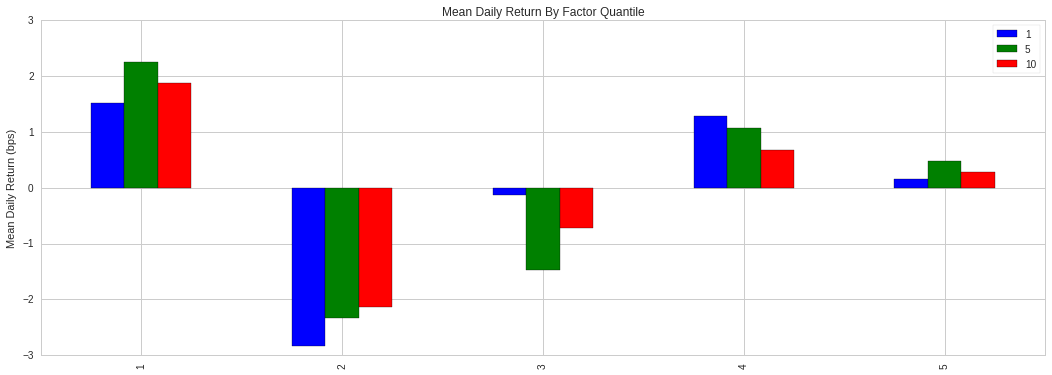By looking at the mean daily return by quantile we can get a real look at how well the factor differentiates forward returns across the signal values. Obviously we want securities with a better signal to exhibit higher returns. For a good factor we'd expect to see negative values in the lower quartiles and positive values in the upper quantiles.

In :
alphalens.plotting.plot_quantile_returns_violin(mean_return_by_q_daily);

/usr/local/lib/python2.7/dist-packages/matplotlib/__init__.py:892: UserWarning: axes.color_cycle is deprecated and replaced with axes.prop_cycle; please use the latter.
warnings.warn(self.msg_depr % (key, alt_key))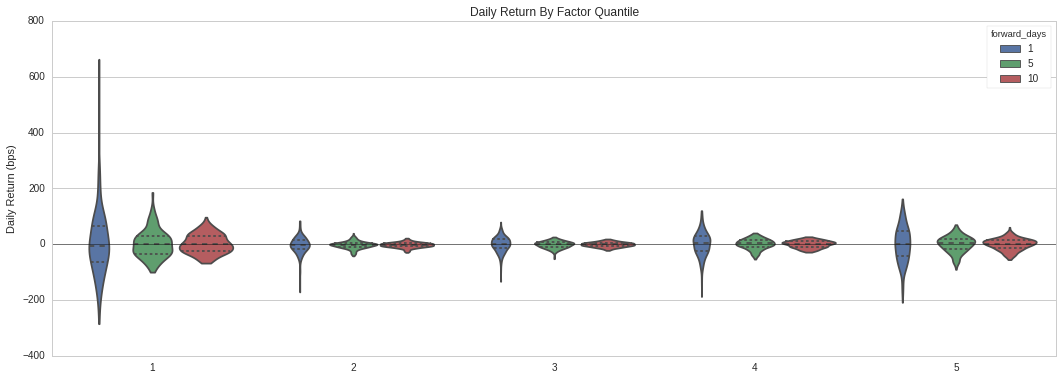This violin plot is similar to the one before it but shows more information about the underlying data. It gives a better idea about the range of values, the median, and the inter-quartile range. What gives the plots their shape is the application of a probability density of the data at different values.

In :
quant_return_spread, std_err_spread = alphalens.performance.compute_mean_returns_spread(mean_return_by_q_daily, 5, 1, std_err)

In :
alphalens.plotting.plot_mean_quantile_returns_spread_time_series(quant_return_spread, std_err_spread);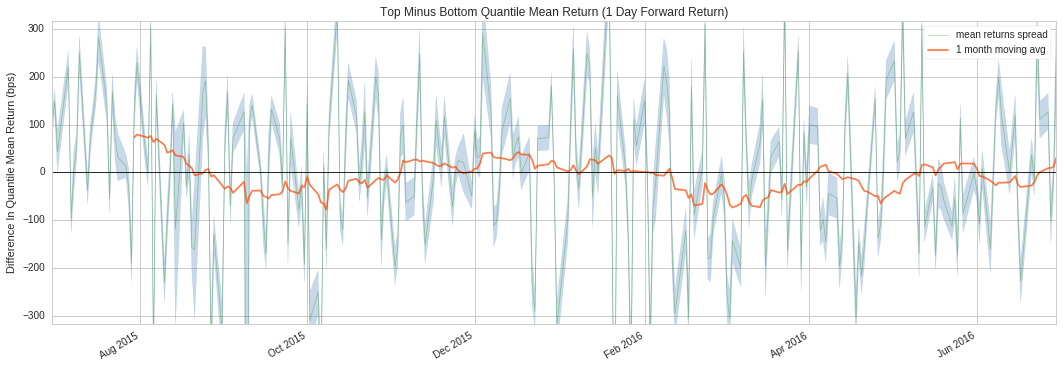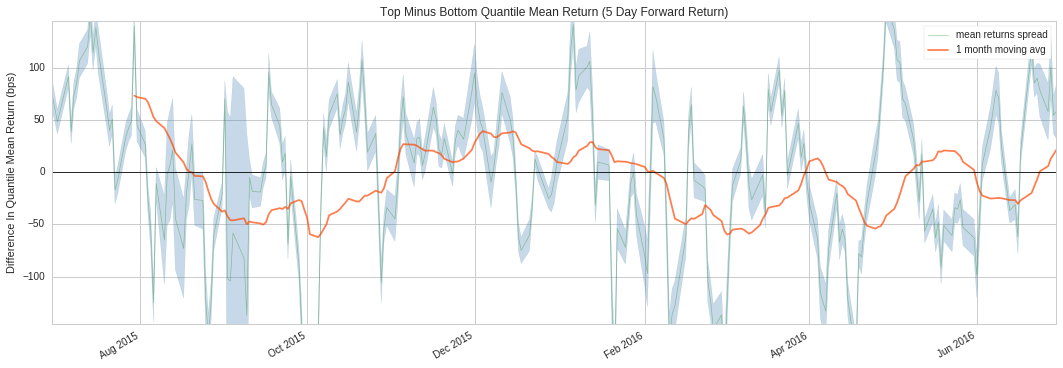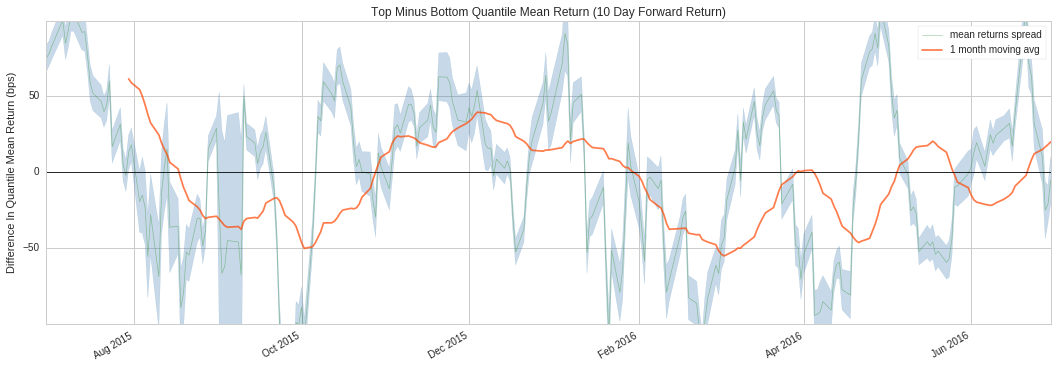This rolling forward returns spread graph allows us to look at the raw spread in basis points between the top and bottom quantiles over time. The green line is the daily returns spread while the orange line is a 1 month average to smooth the data and make it easier to visualize.

In :
alphalens.plotting.plot_cumulative_returns_by_quantile(mean_return_by_q_daily);